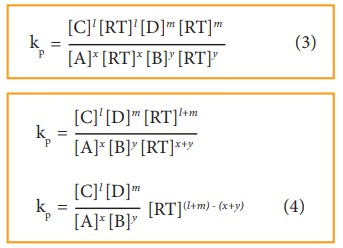Home | | Chemistry 11th std | Relation between Kp and Kc

# Relation between Kp and Kc

Let us consider the general reaction in which all reactants and products are ideal gases.

Relation between Kp and Kc

Let us consider the general reaction in which all reactants and products are ideal gases.The ideal gas equation isSince

Active mass = molar concentration = n/V

P = active mass × RT

Based on the above expression the partial pressure of the reactants and products can be expressed as,

pAx = [A]x [RT]x

pBy = [B]y [RT]y

pC1 = [C]l [RT]l

pmD = [D]m [RT]m

On substitution in Eqn. 2,By comparing equation (1) and (4), we getwhere,

Δng is the difference between the sum of number of moles of products and the sum of number of moles of reactants in the gas phase.

The following relations become immediately obvious.

When Δng = 0

Kp = Kc (RT)0 = Kc

## Example:

H2(g) + I2(g)  2HI (g)

N2 (g) +O2 (g)  2NO(g)

When Δng = +ve

Kp = Kc (RT)+ve

Kp>Kc

2NH3(g)  N2 (g) + 3H2 (g)

PCI5 (g)  PCl3 (g) + Cl2 (g)

When Δng = -ve

Kp = Kc (RT)-ve

Kp< Kc

## Example:

2H2(g) + O2(g)  2H2O (g)

2SO2(g) + O2(g)  2SO3 (g)

## Table 8.1 Relation between equilibrium constants for some reversible reactionsStudy Material, Lecturing Notes, Assignment, Reference, Wiki description explanation, brief detail
11th Chemistry : UNIT 8 : Physical and Chemical Equilibrium : Relation between Kp and Kc |